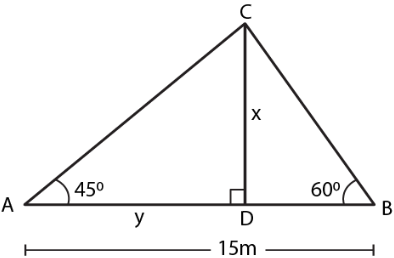Guru

# The angle of elevation of a pillar from a point A on the ground is 45 and from a point B diametrically opposite to A and on the other side of the pillar is 60. Find the height of the pillar, given that the distance between A and B is 15 m.

• 0

sir this is the important  question from the book -ML aggarwal( avichal publication) class 10th , chapter20 , heights and distances
The angle of elevation of a pillar from a point A on the ground is 45
and from a point B diametrically opposite to A
and on the other side of the pillar is 60.
Find the height of the pillar,
given that the distance between A and B is 15 m.

question no 27 , heights and distances , ICSE board

Share

1. Consider CD as the pillar of x m

Angles of elevation of points A and B are 450 and 600It is given that

AB = 15 m

DB = 15 – y

Substituting the values

tan 450 = x/y

So we get

1 = x/y

x = y …… (1)

In triangle CDB

tan 600 = x/(15 – y)

Substituting the values

√3 = x/(15 – y)

So we get

x = √3 (15 – y) ….. (2)

Using both the equations

x = √3 (15 – x)

x = 15√3 – √3x

So we get

x + √3x = 15√3

x (1 + √3) = 15√3

x = 15√3/ (1 + √3)

We can write it as

x = (15 × 1.732)/ (1 + 1.732)

x = 25.98/2.732

x = 9.51

Hence, the height of the pillar is 9.51 m.

• 1